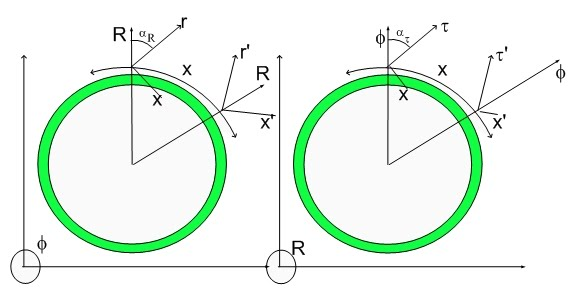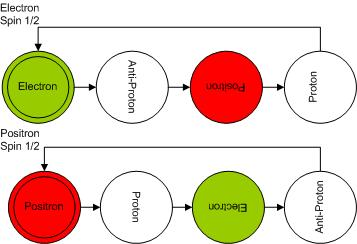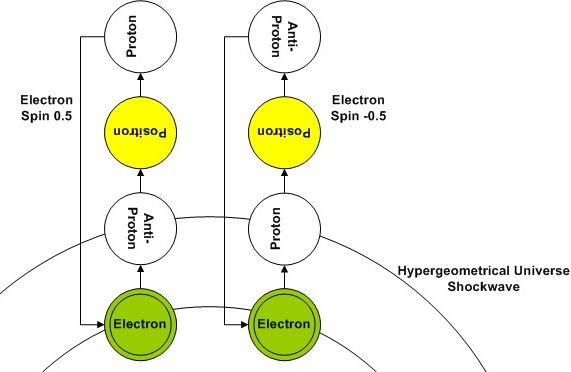# Energy Conservation and the Fundamental Dilatorand

David Freiberg has left a new comment on your post "The Hypergeometrical Force":
Wait, wait, wait. How does a proton, with hundreds of times more mass than an electron, turn into an electron? Does the Law of Conservation of Matter and Energy still apply? If so, where does all the energy go when it does this go and where does the energy come from to add the mass?
David was kind to ask how energy conservation resists such an outrageous transformation as proposed in the Fundamental Dilator paradigm.

The answer has to contain the circumstances where these transformations take place and the emphasis on the fact that the Fundamental Dilator replaces the construct particle.  The replacement of a particle by a coherence between deformation states of a 4D spatial metric (5D spacetime metric) is basic for the understanding of this paradigm.

Currently David thinks about particles (electron, proton..) as having mass, charge - fundamental properties assigned for the life of the particle...:)  Of course, the Fundamental Dilator makes these properties transients associated with states of a coherence.  The only way this would be consistent with observation would be if we were always observing a single face (state) of this coherence.

That is exactly the case. To achieve that one has to remember that the coherence (tunneling between deformation states) is accompanied by spinning within the 4D spatial manifold where the lightspeed expanding hyperspherical shockwave Universe (our 3D Universe) exists.The Ball Diagram shown above displays the not only the transformation (tunneling between deformation states) but also the orientation change (with respect to our 3D Universe) as the dilator goes about his life.

The initial state is the one that is in phase with the 3D Shockwave Universe. Due to the very, very small dimension along one of the directions (R), as the spinning takes place, the overlap between our dilator displacement volume and the 3D Universe vanishes.  Only at "quantized" phases of spinning is that there is overlap. ONLY at "quantized" phases there is interaction.

This stroboscopic nature and the 4D topology are the source of Quantum Mechanics.This diagram shows two electrons belonging to a Cooper Pair.  As you can see, despite of the non-static nature of the dilator, their interaction when the dilator is flush with the 3D shockwave universe, is always consistent with what David considers their nature to be, that is, an electron always repels another electron.

The sideways phases have little overlap with our 3D Universe due to the initial symmetry of the problem and the symmetry of the involved states.

Notice that due to the coherent form in which these changes take place, the dilaton field is generated coherently, that is, the dilaton phases are always in phase with the propagating metric disturbances (dilatons) since they travel at the same speed (the speed of light).  As an aside, only in the case of Cooper pairs, the the intermediate phases anihilate each other's contributions, reducing their interaction to an Anti-Gravitational strength!!!

The Energy conservation is related to the size (3D volume overlap) of this footprint.  Local deformations of the Fabric of Space (local tilts) would impact into the dilator Kinetic Energy or Potential Kinetic Energy (Strong Force). Depending upon what is the choice for the laws of motion (Newton's Laws or my Quantum Lagrangian Principle) mass will behave in a hyperbolic manner (e.g. Lorentz Transform or Strict Relativity - hyperbolic cosines projections) or in a linear manner (through cosine projections).

There is a principle of 4D displacement volume conservation which is applied to the whole Universe, yielding ZERO, that is, the total displacement volume of all dilators in the Universe is ZERO.

The 3D projection of this law is our energy-momentum conservation law.

Cheers,

MP

Currently unrated

### New Comment

required

required (not published)

optional

required